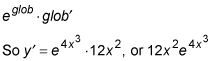##### Differential Equations For DummiesThe chain rule is probably the trickiest among the advanced derivative rules, but it's really not that bad if you focus clearly on what's going on.

Most of the basic derivative rules have a plain old x as the argument (or input variable) of the function. For example,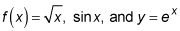all have just x as the argument.

When the argument of a function is anything other than a plain old x, such as y = sin (x2) or ln10x (as opposed to ln x), you've got a chain rule problem.

Here's what you do. You simply apply the derivative rule that's appropriate to the outer function, temporarily ignoring the not-a-plain-old-x argument. Then multiply that result by the derivative of the argument. That's all there is to it.

With chain rule problems, never use more than one derivative rule per step. In other words, when you do the derivative rule for the outermost function, don't touch the inside stuff! Only in the next step do you multiply the outside derivative by the derivative of the inside stuff.

## Practice questions

1. Find the following derivative.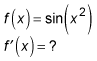2. Find the following derivative.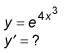1. Using the chain rule: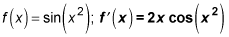Because the argument of the sine function is something other than a plain old x, this is a chain rule problem. Just use the rule for the derivative of sine, not touching the inside stuff (x2), and then multiply your result by the derivative of x2.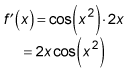2. Using the chain rule: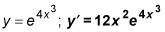The derivative of ex is ex, so by the chain rule, the derivative of eglob is